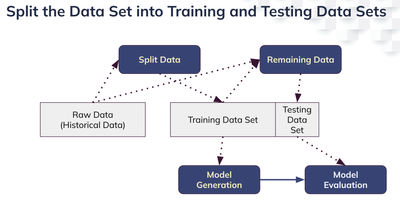cancel
Showing results for
Did you mean:

## Split a Dataset Into Training and Testing Data SetsEmployee

## Introduction

The train-test split procedure is used to evaluate the performance of machine learning (ML) algorithms. As part of data preparation for machine learning, you may need to split a dataset into training and testing sets.

Build Machine Learning Models using Incorta Materialized View

## Applied to

• On-Premises versions 4.9.5+ or later

## Let's Go

In Incorta, splitting raw data into two data sets, a training data set and a testing data det, involves two materialized views (MVs).### Training Data Set

A training data set is used to fit a machine learning model. You can use the randomSplit function to split a data set into training data and testing data sets, then you can save the training data set as an Incorta materialized view.

Note in the example below, you specify the split percentage when invoking the function.

```df = read('SCHEMANAME.TABLENAME')

#weights for splits, sum no more than 1train_df,test_df = df.randomSplit([0.7, 0.3])save(train_df)```

### Testing Data Set

A test data set is used to evaluate the fitted machine learning model. Read the training data set first that you just created above, then use the exceptAll function to get the rest of the raw data as the test data set. As a final step, save the result as another Incorta materialized view.

`df = read('SCHEMANAME.TABLENAME')df_train = read('SCHEMANAME.TRAININGDATASET')df_test = df.exceptAll(df_train)save(df_test)`

## Multi-class classification data

A multi-class classification problem involves more than two classes, for example, assigning salesreps to different sales opportunities or classifying support issues into different categories.

A machine larning model cannot be trained for categorizing a label if the label does not exist in the training data set.  If you use random split, it is likely that some labels may not be included in the training data set.

Here is a function that can be used to split the data set with multi-class classification data to ensure the sampling process will include all labels in the training data.

```from pyspark.sql.functions import lit

def stratified_split(input_df, frac, label_col, seed=10) :    """ Stratified split of a dataframe into training and testing set.
return training only
"""    fractions = input_df.select(label_col).distinct().withColumn("fraction", lit(frac)).rdd.collectAsMap()    df_train = input_df.stat.sampleBy(label_col, fractions, seed)    return df_train```

This function will split the labels proportionally between the training and test data sets.

```df = read('<SCHEMANAME>.<TABLENAME>')

### train_df,test_df = df.randomSplit([0.7, 0.3])train_df = stratified_split(df, 0.7, "<LABELCOLNAME>" )save(train_df)```
Best Practices IndexJust here to browse knowledge? This might help!

Contributors
Version history
Last update:
‎10-24-2022 03:19 AM
Updated by: